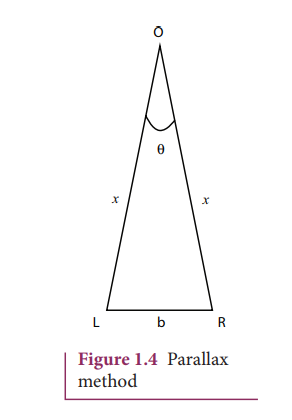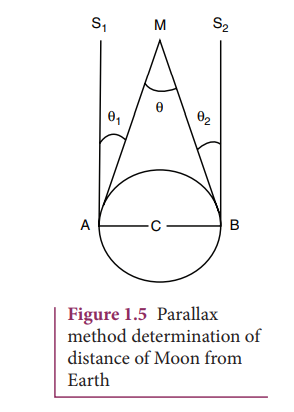Home | | Physics 11th std | Solved Example Problems for Measurement of length

# Solved Example Problems for Measurement of length

Physics : Nature of Physical World and Measurement

## Solved Example Problems for Measurement of length

Example 1.1

From a point on the ground, the top of a tree is seen to have an angle of elevation 60°. The distance between the tree and a point is 50 m. Calculate the height of the tree?

## Solution

Angle θ = 60°

The distance between the tree and a point = 50 m

Height of the tree (h) = ?

For triangulation method tan

h = x tan θ

= 50 × tan 60°

= 50 × 1.732

h = 86.6 mThe height of the tree is 86.6 m.

## Parallax method

Very large distances, such as the distance of a planet or a star from the Earth can be measured by the parallax method. Parallax is the name given to the apparent change in the position of an object with respect to the background, when the object is seen from two different positions. The distance between the two positions (i.e., points of observation) is called the basis (b). For example, consider Figure 1.4., an observer is specified by the position O. The observer is holding a pen before him, against the background of a wall. When the pen is looked at first by our left eye L (closing the right eye) and then by our right eye R (closing the left eye), the position of the pen changes with respect to the back ground of the wall. The shift in the position of an object (say, a pen) when viewed with two eyes, keeping one eye closed at a time is known as Parallax. The distance between the left eye (L) and the right eye (R) in this case is the basis.

LOR is called the parallax angle or parallactic angle.

Taking LR as an arc of length b and radius LO = RO = x

we get θ = b/x, b-basis, x-unknown distance

Knowing ‘b’ and measuring θ, we can calculate x.## Determination of distance of Moon from Earth

In Figure 1.5, C is the centre of the Earth. A and B are two diametrically opposite places on the surface of the Earth. From A and B, the parallaxes θ1 and θ2 respectively of Moon M with respect to some distant star are determined with the help of an astronomical telescope. Thus, the total parallax of the Moon subtended on Earth AMB = θ1 + θ2 = θ.

If  θ  is  measured  in  radians,  thenKnowing  the values of AB and θ,

we can calculate the distance MC of Moon from the Earth.Example 1.2

The Moon subtends an angle of 1° 55 at the base line equal to the diameter of the Earth. What is the distance of the Moon from the Earth? (Radius of the Earth is 6.4 × 106 m)

SolutionRadius of the Earth = 6.4 × 106 m

From the Figure 1.5 AB is the diameter of the Earth (b)= 2 × 6.4 × 106 m Distance of the Moon from the Earth x = ?The word RADAR stands for radio detection and ranging. A radar can be used to measure accurately the distance of a nearby planet such as Mars. In this method, radio waves are sent from transmitters which, after reflection from the planet, are detected by the receiver. By measuring, the time interval (t) between the instants the radio waves are sent and received, the distance of the planet can be determined aswhere v is the speed of the radio wave. As the time taken (t) is for the distance covered during the forward and backward path of the radio waves, it is divided by 2 to get the actual distance of the object. This method can also be used to determine the height, at which an aeroplane flies from the ground.Example 1.3

A RADAR signal is beamed towards a planet and its echo is received 7 minutes later. If the distance between the planet and the Earth is 6.3 × 1010 m. Calculate the speed of the signal?

Solution

The distance of the planet from the Earth d = 6.3 × 1010 mThe speed of signalStudy Material, Lecturing Notes, Assignment, Reference, Wiki description explanation, brief detail
11th Physics : UNIT 1 : Nature of Physical World and Measurement : Solved Example Problems for Measurement of length |